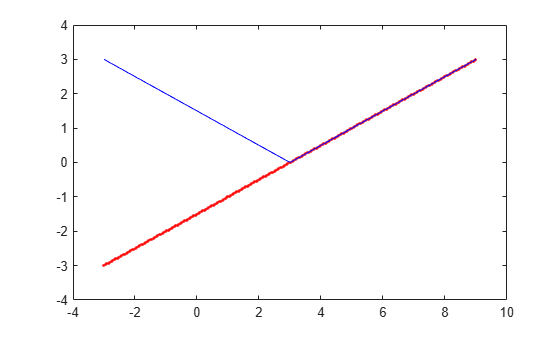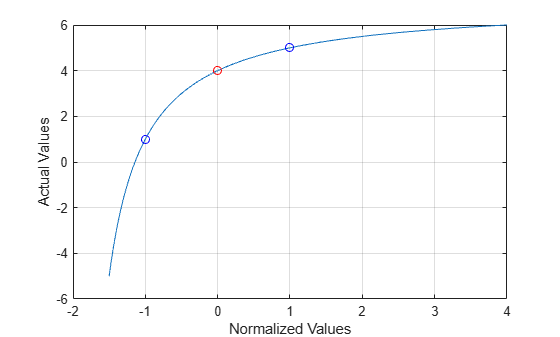# actual2normalized

Transform actual values to normalized values

## Syntax

```NV = actual2normalized(uElement,AV) [NV,ndist] = actual2normalized(uElement,AV) ```

## Description

`NV = actual2normalized(uElement,AV)` transforms the values `AV` of the uncertain element `uElement` into normalized values `NV`. If `AV` is the nominal value of `uElement`, `NV` is 0. Otherwise, `AV` values inside the uncertainty range of `uElement` map to the unit ball `||NV|| <= 1`, and values outside the uncertainty range map to `||NV|| > 1`. The argument `AV` can contain a single value or an array of values. `NV` has the same dimensions as `AV`.

```[NV,ndist] = actual2normalized(uElement,AV)``` also returns the normalized distance `ndist` between the values `AV` and the nominal value of `uElement`. This distance is the norm of `NV`. Therefore, `ndist <= 1` for values inside the uncertainty range of `uElement`, and `ndist > 1` for values outside the range. If `AV` is an array of values, then `ndist` is an array of normalized distances.

The robustness margins computed by`robstab` and `robgain` serve as bounds for the normalized distances in `ndist`. For example, if an uncertain system has a stability margin of 1.4, this system is stable for all uncertain element values whose normalized distance from the nominal is less than 1.4.

## Examples

collapse all

For uncertain real parameters whose range is symmetric about their nominal value, the normalized distance is intuitive, scaling linearly with the numerical difference from the uncertain real parameter's nominal value.

Create uncertain real parameters with a range that is symmetric about the nominal value, where each end point is 1 unit from the nominal. Points that lie inside the range are less than 1 unit from the nominal, while points that lie outside the range are greater than 1 unit from the nominal.

```a = ureal('a',3,'range',[1 5]); NV = actual2normalized(a,[1 3 5])```
```NV = 1×3 -1.0000 0 1.0000 ```
`NV = actual2normalized(a,[2 4])`
```NV = 1×2 -0.5000 0.5000 ```
`NV = actual2normalized(a,[0 6])`
```NV = 1×2 -1.5000 1.5000 ```

Plot the normalized values and normalized distance for several values.

```values = linspace(-3,9,250); [nv,ndist] = actual2normalized(a,values); plot(values,nv,'r.',values,ndist,'b-')```Create a nonsymmetric parameter. The end points are 1 normalized unit from nominal, and the nominal is 0 normalized units from nominal. Moreover, points inside the range are less than 1 unit from nominal, and points outside the range are greater than 1 unit from nominal. However, the relationship between the normalized distance and numerical difference is nonlinear.

```au = ureal('ua',4,'range',[1 5]); NV = actual2normalized(au,[1 4 5])```
```NV = 1×3 -1 0 1 ```
`NV = actual2normalized(au,[2 4.5])`
```NV = 1×2 -0.8000 0.4000 ```
`NV = actual2normalized(au,[0 6])`
```NV = 1×2 -1.1429 4.0000 ```

Graph the relationship between actual and normalized values. The relationship is very nonlinear.

```AV = linspace(-5,6,250); NV = actual2normalized(au,AV); plot(NV,AV,0,au.NominalValue,'ro',-1,au.Range(1),'bo',1,au.Range(2),'bo') grid, xlabel('Normalized Values'), ylabel('Actual Values')```The red circle shows the nominal value (normalized value = 0). The blue circles show the values at the edges of the uncertainty range (normalized values = -1, 1).

## Algorithms

For details on the normalize distance, see Normalizing Functions for Uncertain Elements.

## Version History

Introduced before R2006a